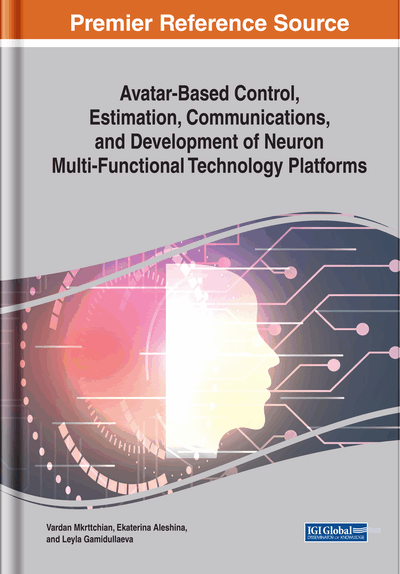# Improving Algorithms for Learning Radial Basic Functions Networks to Solve the Boundary Value Problems

DOI: 10.4018/978-1-7998-1581-5.ch004
OnDemand:
(Individual Chapters)
Available
\$37.50
No Current Special Offers

## Abstract

Digital twins are widely used in modern industry. A digital twin is a computer model that copies the behavior of a physical object. Digital twins of objects with distributed parameters are mathematically boundary value problems for partial differential equation. Traditionally, such problems are solved by finite difference and finite element methods, which require a complex grid construction procedure. The numerical solution of boundary value problems employs mesh less methods that do not require grid construction. Among mesh fewer methods, projection methods that use radial basis functions (RBFs) as basic functions are popular. Methods using RBF allow us to obtain a differentiable solution at any point in the solution domain, applicable to problems of arbitrary dimension with complex computational domains. When solving the problem, the parameters of the basic functions are selected, and the weights are calculated so that the residuals obtained after the substitution of the approximate solution at the test points in the equation are zero.
Chapter Preview
Top

## Introduction

Digital twins are widely used in modern industry (Grieves, 2014;Uhlemann, et al., 2017;Madni, et al., 2019). The digital twin is a dynamic virtual model of a system, process or service. The digital twin continuously learns and updates its parameters, receiving information from a variety of sensors, correctly representing the state of a physical object. When learning, they use current data from sensors, from control devices, from the external environment; it combines actual data with the knowledge gained from specialists in this field. Digital twins allow you to monitor systems and processes in real time and analyze data in a timely manner to prevent problems before they occur, schedule preventive maintenance, reduce downtime, open up new business opportunities and plan future updates and new developments. Digital twins of objects with distributed parameters are mathematically boundary value problems for partial differential equations. (PDE) (Farlow, 1993).

When modeling complex technical objects, software packages based on the finite element method or finite differences are usually used (Mazumder, 2015). However, modeling a real object with their help runs up against a number of fundamental difficulties (Vasilyev, et al., 2018). First, accurate information about differential equations describing the behavior of an object is usually absent due to the complexity of describing the processes occurring in it. Second, to apply the finite element and finite difference methods, one needs to know the initial and boundary conditions, information about which is usually incomplete and inaccurate. Thirdly, during the operation of a real object, its properties and characteristics, the parameters of the processes occurring in it can change. This requires appropriate adaptation of the model, which is difficult to carry out with models built on the basis of finite element methods and finite differences.

An alternative to finite difference and finite element methods is meshless methods (Belytschko, et al., 1996; Liu, 2003; Griebel and Marc, 2008), most of which are projection methods. These methods give an approximate analytical solution in the form of a sum of basis functions multiplied by weights. Radial basis functions (RBF) are popular as basic functions (Kansa, 1999;Buhmann, 2004;Fasshauer, 2007;Chen and Fu, 2014). Methods using RBF allow to obtain a differentiable solution at an arbitrary point in the solution domain as a function satisfying the required smoothness conditions, are universal, allow working with complex geometry of computational domains, are applicable to solving problems of any dimension. Methods based on RBF require, for the selected parameters of the radial basis functions, finding the weight vector, so that the obtained approximate solution provides, with an admissible error, the equation and boundary conditions on a certain set of sampling points. For example, the sum of the squares of the residuals at the sampling points should be small. The main disadvantage of using RBF is the need for an unformalized selection of parameters of basic functions.

## Complete Chapter List

Search this Book:
Reset